Elastic Model of SLCD Holding Power

# An Elastic Model of the Holding Power of Spring Loaded Camming Devices Used as Rock Climbing Anchors

## Dave Custer

#### html markup by Susan Ruff

```

```
As a basis for specifications for the testing of spring loaded camming devices used as rock climbing anchors, two successive models of these devices are developed. The rigid body model assumes an infinitely strong, undeformable cam; it determines the cam's basic shape and the relationship between this shape and the cam's frictional holding ability. Based on the rigid body model, the second, elastic model takes deformation and material strength into account; this elastic model determines the relationship between cam shape and the shear strength of the cam. The models predict two failure modes for camming devices placed in vertical, parallel cracks: inadequate friction and shear yield failure. A measure of the limitations imposed by these failure modes would provide climbers with information necessary for intelligent anchor placement.

### Introduction

Spring loaded camming devices (SLCDs) are a type of anchor that rock climbers use to connect themselves to cracks or other irregularies in a rock cliff. These devices work through a combination of the wedge principle and friction: once the cams are placed in a crack, any force pulling the cams down causes the cams to press tightly against the crack walls, generating a frictional force that retards the downward pull. Figure 1 (80K) shows a typical SLCD placed in a test fixture crack. Springs built into the device (not visible) keep the cams snug against the walls. Any downward load applied at the carabiner rotates the cams and forces them against the walls, effectively wedging the device more tightly in place. Over the past two decades, SLCDs have proven to be an effective anchor, and now SLCDs are manufactured in a variety of sizes, shapes, configurations, and constructions.

The current methods of testing the spectrum of available SLCDs are based either on anecdotal evidence that lacks an experimental control or on pull-to-failure tests that determine the maximum force obtainable before the device collapses. Such tests provide neither a full nor accurate picture of a device's holding ability. As a first step toward developing better methods for the testing of SLCDs, this report develops a mathematical model for how SLCDs work, suggests theoretical limitations of SLCD performance determined by the model, shows preliminary verification of this model, and suggests implications for both cam testing and the use of SLCDs.

### Modeling SLCDs in Parallel Cracks

A succession of two models is used to develop an understanding of SLCD performance in parallel cracks. The rigid model assumes that the actual materials that compose the cams are perfect: perfectly rigid and strong. This rigid model provides a basic theory of why camming devices work, defines the cam's shape, and determines the relationship between the shape and the friction coefficient. The elastic model builds on the rigid model by additionally taking elasticity and shear yield into account. The elastic model provides estimates of cam deformation, the resulting shear loading, and the maximum applied force to failure.

These models are developed for the condition shown in Figure 1, an SLCD subject to a downward applied force while placed in a vertical, parallel crack. The parallel crack constraint is chosen for two reasons. From the point of view of the climber, any evaluation of these devices must address performance in parallel cracks because SLCDs are designed explicitly for use in such a situation. The parallel crack criterion is also important because it bounds narrowing and flaring cracks; parallel crack results define a performance limit for each regime.

#### The Rigid Model -- Explaining How Camming Devices Work

As a first approximation, a camming device can be modeled as a rod wedged or ``cammed'' in a parallel crack as shown in Figure 2a below. If the rod does not slip out, a downward tug or force applied to the upper end wedges the rod harder into the crack, causing the rod to push against the walls with a normal force. Whether or not the rod slips out is determined by a third force, that of friction. Figure 2b shows the forces on the rod; the friction force on the upper left end of the rod is intentionally omitted. For camming devices that rely on friction, this upper left end corresponds to an internal axle (see Figure 1 or Figure 4) and thus experiences negligible friction.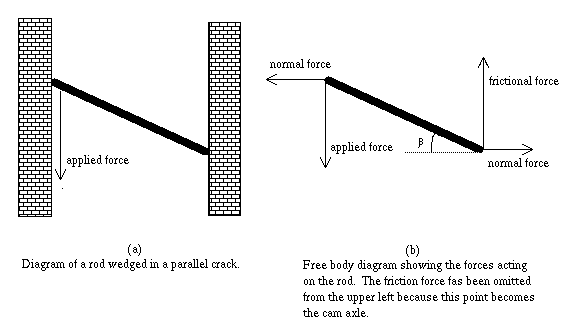If the rod shown in Figure 2 does not move, both the forces and torques must each sum to zero. These equalities and the definition of the friction force yield four equations that define the relation between the applied, normal and friction forces. R is the rod length,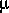is the friction coefficient, and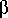is the angle the rod makes with the horizontal.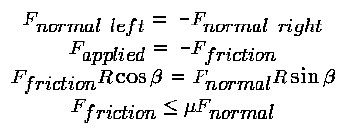Two important results are derived from algebraic manipulation of these equations:Equation 1 shows the wedging effect that multiplies the applied force by a factor of 1/tan. This relationship between the applied and normal forces is invoked in the elastic model to determine cam compression. Equation 2 shows that the maximum camming angle,, at which the rod can remain in the crack is limited by the coefficient of friction,between the rod and the crack surface. For an aluminum rod in a granite crack, a measured value for the friction coefficient is .38, and the corresponding maximum camming angle is about 20 degrees; a rod tipped more than 20 degrees from horizontal would slip out of a granite crack.

To produce a camming device that works over a wide range of crack sizes, multiple rods are combined into a two dimensional surface in the shape of a cam. This shape satisfies the criterion shown in Figure 3a below: the angle between the surface and the line perpendicular to the radius must remain a constant, the camming angle,. In polar coordinates, such a shape is defined by: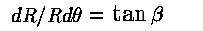Rearranging and integrating yields: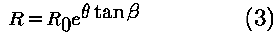Equation 3 defines the logarithmic spiral plotted in Figure 3b. A section cut from this spiral produces the cam (Figure 3c). Typically three or four such cams are mounted together on an axle to produce a complete SLCD.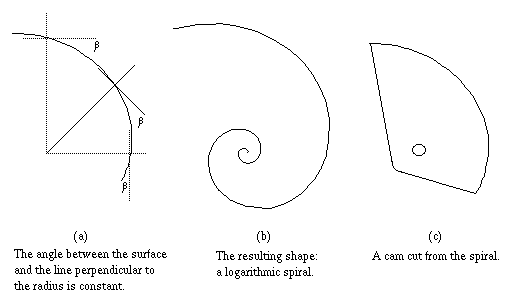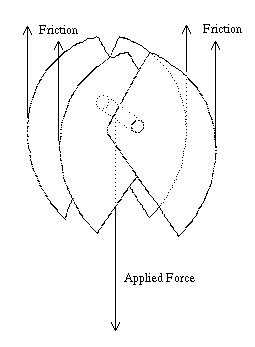Figure 4 shows the forces on a complete SLCD in a parallel crack. The free body diagram is very similar to the rod in Figure 2, though now the contact forces are spread out evenly over all four cams. The camming angle is set by the tightness of the spiral rather than by the rod length and crack width. The relationship between the camming angle and the friction coefficient is still determined by Equation 2: any given cam can only hold if tan<. If the friction coefficient between the cam and the rock surface drops below tan, the device fails due to a lack of frictional holding ability.

#### The Elastic Model -- Materials Failure Modes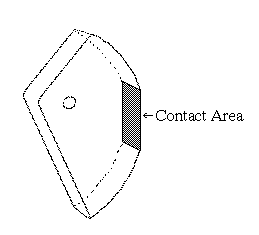Because the rigid model presumes that the cams are perfectly strong and do not bend or deform when weighted, it does not take into account the cam material properties: Young's Modulus, Poisson's Ratio, and shear yield strength. The elastic model assumes the cam shape determined by the rigid model but deforms this shape elastically under an applied force. This deformation produces a contact area as shown in Figure 5. The size of this contact area determines how large the applied force can become before the cam's shear yield strength is exceeded and the device fails.

An approximate size of this contact area can be obtained by applying Hertz's theory2 of the contact between two elastic bodies.Because this equation is derived for cylinders, it only approximates the deformations of cams. Five simplifications may not reflect actual cam geometries:

• Only the compressive (normal) force is taken into account; the applied force is not addressed. 3
• The logarithmic curvature of the cam can be approximated as the curvature of a circle. 4
• The cam is arbitrarily wide relative to the radius W >> R; in typical cams, W and R are of the same order of magnitude.
• The cam has no holes or teeth; manufactured devices are often ``drilled out'' to reduce weight and have teeth to provide ``bite.''
• The effects of plastic deformation are not accounted for.
Given these simplifications, Equation 4 approximates the relationship between the applied force, the geometry, and the material properties: the contact area is proportional to the square root of the compression force, the radius, and the width. While the resulting area is not exact, Equation 4 can be used to estimate the shear stress that develops at the contact area.

Given the contact area from Equation 4, the shear stress at the cam/crack boundary is approximately Fapplied / Acontact. This approximation assumes that the contact forces are evenly distributed over the contact area. The Hertzian contact theory predicts that the distribution is not even but that the maximum stresses are within a factor of 4/pi of the average value.5 Given the shear yield of the material, tau, the contact area from Equation 4, and the relationship between the applied and normal forces (from Equation 1), the maximum force that can be applied to the cam before it shears out is given by:Because cam geometries and material properties can all vary between different SLCDs, the maximum applied force can in turn vary greatly. Figure 6 below shows the estimates of the maximum applied force for a range of cam geometries and materials. The two surfaces represent parameters taken from two popular brands of SLCDs; the upper surface is a 7075 aluminum with a 12.75 degree camming angle and the lower surface is a 6061 aluminum with a 15.00 degree camming angle.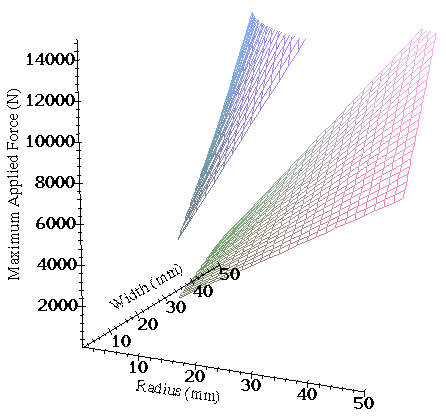Figure 6: Estimated maximum applied force sustainable for two types of SLCDs.

These estimates do not necessarily reflect actual performance; rather, the force estimates are plotted to show the wide range of maximum forces that devices might be expected to sustain. These force estimates span the range of forces that climbers generate. The highest force that a climber can expect is about 12,000N and a climber hanging from an anchor applies a force of about 1500N. According to the elastic model, larger SLCDs can sustain larger forces and the smaller devices are expected to fail under conditions commonly experienced by climbers. Even though several estimations and simplifications are incorporated into the elastic model, the range of performance expectations remains large. The lower end of the applied force range is of particular importance because the smaller devices may fail under nominal conditions.

### Implications of the Model

The perfect SLCD is light weight and durable, fits in a wide range of crack sizes, anchors reliably to the most slippery rock, has a narrow profile (width) that allows placement in shallow cracks, and can sustain the largest applied forces a falling climber can exert. These optimal characteristics can only be obtained through variation of the cam's geometry and material properties: the camming angle, radius, width, Young's Modulus, Poisson's Ratio, and the shear yield. Every cam is a compromise; efforts to lighten or narrow the cam reduce both durability and maximum load, and greater range is traded for less frictional holding ability. These compromises are implicit in Equation 2, which constrains the camming angle to be less than the arctan of the friction coefficient, and Equation 5, which defines the maximum load that can be applied before the onset of shear failure. Even though these equations provide only estimates of cam performance, the model predicts both the wide variation of performance among different SLCDs and the likelihood that some SLCDs, especially smaller ones, fail at forces commonly produced by falling climbers.

Published measurements of force to shear yield and frictional holding ability would provide information that allows climbers to make intelligent decisions concerning SLCDs. Most importantly, climbers would be aware when devices have dangerously low frictional holding ability or maximum load to shear failure. Of similar importance, an explicit awareness of cam limitations allows climbers to place the devices more securely and with greater confidence. Finally, performance information would provide climbers with a way of comparing SLCDs made by different manufacturers. To date, this information is either not explicitly stated or simply not available to the climbing community. Making performance information available would alert climbers to the existence of SLCD limitations and provide climbers with the information required to successfully work around these limitations.

#### Footnotes

2 from Ball and Roller Bearings, Eschmann, Hasbargen, Weigand, J Wiley and Sons, pg 113.

3 The literature suggests that the effect of the non-compressive force is small (Contact Mechanics, KL Johnson, Cambridge University Press, 1985).

4 Finite element analysis work by Luke Sosnowski suggests a negligible difference between logarithmic and circular curvature.

5 Ball and Roller Bearings, Eschmann, Hasbargen, Weigand, J Wiley and Sons, pg 118.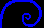Dave Custer's home page
```

```